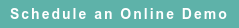# RoyaltyStat Blog

### Posts by Topic

In DuPont’s profit identity, “return on assets” (profit rate) is equal to profit margin multiplied by asset turnover. Using DuPont’s profit formula, combined with an assumption that profit margin and asset turnover (measures of economic performance and adopted technology) are independent, we showed on a prior blog that the coefficient of variation of return on assets is greater than of the profit margin. It follows that profit margin is more reliable (has a lower coefficient of variation) than return on assets. In science, coefficient of variation is an accepted method of determining the reliability of a selected variable.

Here, we expand the short formula of the coefficient of variation of return on assets to show in more detail the algebra involved. We start with several useful definitions and statistical results obtained on a prior blog published on October 22, 2018:

Define the relevant M, R, and T variables and assume that M and T are independent (assume they are not correlated):

(1)  R = [M ∙ T] = asset return (OECD lingo: “return on assets”), where

(2)  M = Profit / Revenue = profit margin

(3)  T = Revenue / Assets = asset turnover

(4)  E[M ∙ T] = µR = µM µT

(5)  Var[M ∙ T] = σR2 = σM2 σT2 + σM2 µT2 + σT2 µM2

(6)  KR2 = (σR2 / µR2) = {σM2 σT2 + σM2 µT2 + σT2 µM2} / µM2 µT2

(7)  KR2 = {KM2 (KT2 + 1) + KT2} > KM2

Now, we expand the coefficient of variation formula (6) to obtain a short version (7):

(6)  KR2 = (σR2 / µR2) = {σM2 σT2 + σM2 µT2 + σT2 µM2} / µM2 µT2

= (σM2 σT2 / µM2 µT2) + (σM2 µT2 / µM2 µT2) + (σT2 µM2 / µM2 µT2)

= KM2 KT2 + KM2 + KT2

(7)  KR2 = {KM2 (KT2 + 1) + KT2},

which shows that KRis greater than KM2 because KT2 is positive.

Takeaway: Under the M and T independence assumption, equation (7) shows that the claimed à priori advantage of return on asset over profit margin can't hold, and we must rely on analysis of facts and circumstances of the audit case.

Published on Oct 23, 2018 2:57:08 AM

Ednaldo Silva (Ph.D.) is founder and managing director of RoyaltyStat. He helped draft the US transfer pricing regulations and developed the comparable profits method called TNNM by the OECD. He can be contacted at: esilva@royaltystat.com

RoyaltyStat provides premier online databases of royalty rates extracted from unredacted license agreements
and normalized company financials (income statement, balance sheet, cash flow). We provide high-quality data, built-in analytical tools, customer training and attentive technical support.Topics: Net Profit Indicator